# Operational amplifier applications

﻿
Operational amplifier applications

This article illustrates some typical applications of operational amplifiers. A simplified schematic notation is used, and the reader is reminded that many details such as device selection and power supply connections are not shown.

## Practical considerations

### Input offset problems

It is important to note that the equations shown below, pertaining to each type of circuit, assume that an ideal op amp is used. Those interested in construction of any of these circuits for practical use should consult a more detailed reference. See the External links and Further reading sections.

Resistors used in practical solid-state op-amp circuits are typically in the kΩ range. Resistors much greater than 1 MΩ cause excessive thermal noise and make the circuit operation susceptible to significant errors due to bias or leakage currents.

Practical operational amplifiers draw a small current from each of their inputs due to bias requirements and leakage. These currents flow through the resistances connected to the inputs and produce small voltage drops across those resistances. In AC signal applications this seldom matters. If high-precision DC operation is required, however, these voltage drops need to be considered. The design technique is to try to ensure that these voltage drops are equal for both inputs, and therefore cancel. If these voltage drops are equal and the common-mode rejection ratio of the operational amplifier is good, there will be considerable cancellation and improvement in DC accuracy.

If the input currents into the operational amplifier are equal, to reduce offset voltage the designer must ensure that the DC resistance looking out of each input is also matched. In general input currents differ, the difference being called the input offset current, Ios. Matched external input resistances Rin will still produce an input voltage error of  Rin·Ios .  Most manufacturers provide a method for tuning the operational amplifier to balance the input currents (e.g., "offset null" or "balance" pins that can interact with an external voltage source attached to a potentiometer). Otherwise, a tunable external voltage can be added to one of the inputs in order to balance out the offset effect. In cases where a design calls for one input to be short-circuited to ground, that short circuit can be replaced with a variable resistance that can be tuned to mitigate the offset problem.

Note that many operational amplifiers that have MOSFET-based input stages have input leakage currents that will truly be negligible to most designs.

### Power supply effects

Although the power supplies are not shown in the operational amplifier designs below, they can be critical in operational amplifier design.

Power supply imperfections (e.g., power signal ripple, non-zero source impedance) may lead to noticeable deviations from ideal operational amplifier behavior. For example, operational amplifiers have a specified power supply rejection ratio that indicates how well the output can reject signals that appear on the power supply inputs. Power supply inputs are often noisy in large designs because the power supply is used by nearly every component in the design, and inductance effects prevent current from being instantaneously delivered to every component at once. As a consequence, when a component requires large injections of current (e.g., a digital component that is frequently switching from one state to another), nearby components can experience sagging at their connection to the power supply. This problem can be mitigated with copious use of bypass capacitors connected across each power supply pin and ground. When bursts of current are required by a component, the component can bypass the power supply by receiving the current directly from the nearby capacitor (which is then slowly recharged by the power supply).

Additionally, current drawn into the operational amplifier from the power supply can be used as inputs to external circuitry that augment the capabilities of the operational amplifier. For example, an operational amplifier may not be fit for a particular high-gain application because its output would be required to generate signals outside of the safe range generated by the amplifier. In this case, an external push–pull amplifier can be controlled by the current into and out of the operational amplifier. Thus, the operational amplifier may itself operate within its factory specified bounds while still allowing the negative feedback path to include a large output signal well outside of those bounds.

## Circuit applications

### Comparator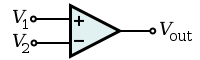Bistable output that indicates which of the two inputs has a higher voltage. That is,$V_{\text{out}} = \begin{cases} V_{\text{S}+} & \text{if } V_1 > V_2, \\ V_{\text{S}-} & \text{if } V_1 < V_2, \end{cases}$

where VS + and VS − are nominally the positive and negative supply voltages (which are not shown in the diagram).

### Inverting amplifier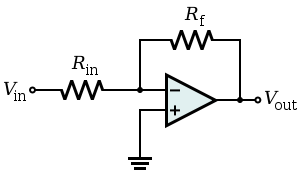An inverting amplifier uses negative feedback to invert (i.e., negate) and amplify a voltage. In particular, the RinRf resistor network acts as an electronic seesaw (i.e., a class-1 lever) where the inverting (i.e., ) input of the operational amplifier is like a fulcrum about which the seesaw pivots. That is, because the operational amplifier is in a negative-feedback configuration, its internal high gain effectively fixes the inverting (i.e., −) input at the same 0 V (ground) voltage of the non-inverting (i.e., +) input, which is similar to the stiff mechanical support provided by the fulcrum of the seesaw. Continuing the analogy,

• Just as the movement of one end of the seesaw is opposite the movement of the other end of the seesaw, positive movement away from 0 V at the input of the RinRf network is matched by negative movement away from 0 V at the output of the network; thus, the amplifier is said to be inverting.
• In the seesaw analogy, the mechanical moment or torque from the force on one side of the fulcrum is balanced exactly by the force on the other side of the fulcrum; consequently, asymmetric lengths in the seesaw allow for small forces on one side of the seesaw to generate large forces on the other side of the seesaw. In the inverting amplifier, electrical current, like torque, is conserved across the RinRf network and relative differences between the Rin and Rf resistors allow small voltages on one side of the network to generate large voltages (with opposite sign) on the other side of the network. Thus, the device amplifies (and inverts) the input voltage. However, in this analogy, it is the reciprocals of the resistances (i.e., the conductances or admittances) that play the role of lengths in the seesaw.

Hence, the amplifier output is related to the input as in$V_{\text{out}} = -\frac{R_{\text{f}}}{R_{\text{in}}} V_{\text{in}}\!\,$.

So the voltage gain of the amplifier is A = − Rf / Rin where the negative sign is a convention indicating that the output is negated. For example, if Rf is 10 kΩ and Rin is 1 kΩ, then the gain is −10 kΩ/1 kΩ, or −10 (or −10 V/V). Moreover, the input impedance of the device is Rin because the operational amplifier's inverting (i.e., −) input is a virtual ground.

In a real operational amplifier, the current into its two inputs is small but non-zero (e.g., due to input bias currents). The current into the inverting (i.e., −) input of the operational amplifier is drawn across the Rin and Rf resistors in parallel, which appears like a small parasitic voltage difference between the inverting (i.e., −) and non-inverting (i.e., +) inputs of the operational amplifier. To mitigate this practical problem, a third resistor of value$R_{\text{f}} \| R_{\text{in}} \triangleq R_{\text{f}} R_{\text{in}} / (R_{\text{f}} + R_{\text{in}})$ can be added between the non-inverting (i.e., +) input and the true ground. This resistor does not affect the idealized operation of the device because no current enters the ideal non-inverting input. However, in the practical case, if input currents are roughly equivalent, the voltage added at the inverting input will match the voltage at the non-inverting input, and so this common-mode signal will be ignored by the operational amplifier (which operates on differences between its inputs).

### Non-inverting amplifierAmplifies a voltage (multiplies by a constant greater than 1)$V_{\text{out}} = V_{\text{in}} \left( 1 + \frac{R_2}{R_1} \right)\,$
• Input impedance$Z_{\text{in}} \approx \infin$
• The input impedance is at least the impedance between non-inverting ( + ) and inverting ( ) inputs, which is typically 1 MΩ to 10 TΩ, plus the impedance of the path from the inverting ( ) input to ground (i.e., R1 in parallel with R2).
• Because negative feedback ensures that the non-inverting and inverting inputs match, the input impedance is actually much higher.
• Although this circuit has a large input impedance, it suffers from error of input bias current.
• The non-inverting ( + ) and inverting ( ) inputs draw small leakage currents into the operational amplifier.
• These input currents generate voltages that act like unmodeled input offsets. These unmodeled effects can lead to noise on the output (e.g., offsets or drift).
• Assuming that the two leaking currents are matched, their effect can be mitigated by ensuring the DC impedance looking out of each input is the same.
• The voltage produced by each bias current is equal to the product of the bias current with the equivalent DC impedance looking out of each input. Making those impedances equal makes the offset voltage at each input equal, and so the non-zero bias currents will have no impact on the difference between the two inputs.
• A resistor of value$R_1 \| R_2 \triangleq \left(\frac{1}{R_1} + \frac{1}{R_2}\right)^{-1} = \frac{ R_1 R_2 }{ R_1 + R_2 },\,$
which is the equivalent resistance of R1 in parallel with R2, between the Vin source and the non-inverting ( + ) input will ensure the impedances looking out of each input will be matched.
• The matched bias currents will then generate matched offset voltages, and their effect will be hidden to the operational amplifier (which acts on the difference between its inputs) so long as the CMRR is good.
• Very often, the input currents are not matched.
• Most operational amplifiers provide some method of balancing the two input currents (e.g., by way of an external potentiometer).
• Alternatively, an external offset can be added to the operational amplifier input to nullify the effect.
• Another solution is to insert a variable resistor between the Vin source and the non-inverting ( + ) input. The resistance can be tuned until the offset voltages at each input are matched.
• Operational amplifiers with MOSFET-based input stages have input currents that are so small that they often can be neglected.

### Differential amplifier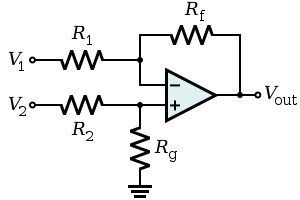The name "differential amplifier" should not be confused with the "differentiator," which is also shown on this page.
The "instrumentation amplifier," which is also shown on this page, is a modification of the differential amplifier that also provides high input impedance.

The circuit shown computes the difference of two voltages multiplied by some constant. In particular, the output voltage is:$V_{\text{out}} = \frac{ \left( R_{\text{f}} + R_1 \right) R_{\text{g}} }{\left( R_{\text{g}} + R_2 \right) R_1} V_2 - \frac{R_{\text{f}}}{R_1} V_1.$

The differential input impedance Zin (i.e., the impedance between the two input pins) is approximately R1 + R2. The input currents vary with the operating point of the circuit. Consequently, if the two sources feeding this circuit have appreciable output impedance, then non-linearities can appear in the output. An instrumentation amplifier mitigates these problems.

• Under the condition that the Rf/R1 = Rg/R2, the output expression becomes:$V_{\text{out}} = A (V_{\text{2}} - V_{\text{1}})\,$   where$A \triangleq \frac{ R_{\text{f}} }{ R_1 }$   is the differential gain of the circuit.
Moreover, the amplifier synthesized with this choice of parameters has good common-mode rejection in theory because components of the signals that have V1 = V2 are not expressed on the output. Although this property is described here with resistances, it is a more general property of the impedances in the circuit. So, for example, if a compensation capacitor is added across any resistor (e.g., to improve phase margin and ensure closed-loop stability of the operational amplifier), similar changes need to be made in the rest of the circuit to maintain the ratio balance. Otherwise, high-frequency components common to both V1 and V2 can express themselves on the output. Additionally, because of leakage or bias currents in a real operational amplifier, it is usually desirable for the impedance looking out each input to the operational amplifier to be equal to the impedance looking out of the other input of the operational amplifier. Otherwise, the same current into each operational amplifier input will generate a parasitic differential signal and thus a parasitic output component. Consequently, choosing R1 = R2 and Rf = Rg is common in practice.
• In the special case when Rf/R1 = Rg/R2, as before, and Rf = R1, the differential gain A = 1, and the circuit is a differential follower with:$V_{\text{out}} = V_2 - V_1.\,$

### Voltage follower (Unity Buffer Amplifier)Used as a buffer amplifier to eliminate loading effects (e.g., connecting a device with a high source impedance to a device with a low input impedance).$V_{\text{out}} = V_{\text{in}} \!\$$Z_{\text{in}} = \infty$ (realistically, the differential input impedance of the op-amp itself, 1 MΩ to 1 TΩ)

Due to the strong (i.e., unity gain) feedback and certain non-ideal characteristics of real operational amplifiers, this feedback system is prone to have poor stability margins. Consequently, the system may be unstable when connected to sufficiently capacitive loads. In these cases, a lag compensation network (e.g., connecting the load to the voltage follower through a resistor) can be used to restore stability. The manufacturer data sheet for the operational amplifier may provide guidance for the selection of components in external compensation networks. Alternatively, another operational amplifier can be chosen that has more appropriate internal compensation.

### Summing amplifier

A summing amplifier sums several (weighted) voltages:$V_{\text{out}} = -R_{\text{f}} \left( \frac{V_1}{R_1} + \frac{V_2}{R_2} + \cdots + \frac{V_n}{R_n} \right)$
• When$R_1 = R_2 = \cdots = R_n$, and Rf independent$V_{\text{out}} = -\frac{R_{\text{f}}}{R_1} ( V_1 + V_2 + \cdots + V_n ) \!\$
• When$R_1 = R_2 = \cdots = R_n = R_{\text{f}}$$V_{\text{out}} = -( V_1 + V_2 + \cdots + V_n ) \!\$
• Output is inverted
• Input impedance of the nth input is Zn = Rn (V is a virtual ground)

### Inverting integrator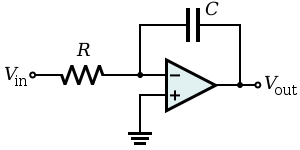Integrates the (inverted) signal over time$V_{\text{out}} = -\int_0^t \frac{ V_{\text{in}} }{RC} \, \operatorname{d}t + V_{\text{initial}}\,$

(where Vin and Vout are functions of time, Vinitial is the output voltage of the integrator at time t = 0.)

• Note that this can also be viewed as a low-pass electronic filter. It is a filter with a single pole at DC (i.e., where ω = 0) and gain.
• There are several potential problems with this circuit.
• It is usually assumed that the input Vin has zero DC component (i.e., has a zero average value). Otherwise, unless the capacitor is periodically discharged, the output will drift outside of the operational amplifier's operating range.
• Even when Vin has no offset, the leakage or bias currents into the operational amplifier inputs can add an unexpected offset voltage to Vin that causes the output to drift. Balancing input currents and replacing the non-inverting ( + ) short-circuit to ground with a resistor with resistance R can reduce the severity of this problem.
• Because this circuit provides no DC feedback (i.e., the capacitor appears like an open circuit to signals with ω = 0), the offset of the output may not agree with expectations (i.e., Vinitial may be out of the designer's control with the present circuit).
Many of these problems can be made less severe by adding a large resistor RF in parallel with the feedback capacitor. At significantly high frequencies, this resistor will have negligible effect. However, at low frequencies where there are drift and offset problems, the resistor provides the necessary feedback to hold the output steady at the correct value. In effect, this resistor reduces the DC gain of the "integrator" – it goes from infinite to some finite value RF / R.

### Inverting differentiator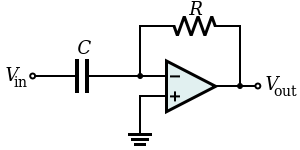Differentiates the (inverted) signal over time.$V_{\text{out}} = -RC \,\frac{\operatorname{d}V_{\text{in}} }{ \operatorname{d}t} \, \qquad \text{where } V_{\text{in}}\text{ and } V_{\text{out}} \text{ are functions of time.}$
• Note that this can also be viewed as a high-pass electronic filter. It is a filter with a single zero at DC (i.e., where angular frequency ω = 0 radians) and gain. The high-pass characteristics of a differentiating amplifier (i.e., the low-frequency zero) can lead to stability challenges when the circuit is used in an analog servo loop (e.g., in a PID controller with a significant derivative gain). In particular, as a root locus analysis would show, increasing feedback gain will drive a closed-loop pole toward marginal stability at the DC zero introduced by the differentiator.

### Instrumentation amplifier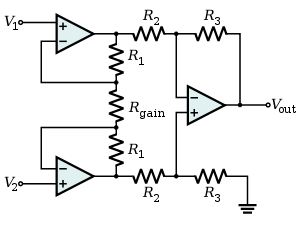Combines very high input impedance, high common-mode rejection, low DC offset, and other properties used in making very accurate, low-noise measurements

### Schmitt trigger

A bistable multivibrator implemented as a comparator with hysteresis.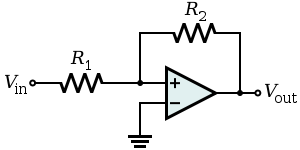In this configuration, the input voltage is applied through the resistor R1 (which may be the source internal resistance) to the non-inverting input and the inverting input is grounded or referenced. The hysteresis curve is non-inverting and the switching thresholds are$\pm \frac{R_1}{R_2}V_{\text{sat}}$ where Vsat is the greatest output magnitude of the operational amplifier.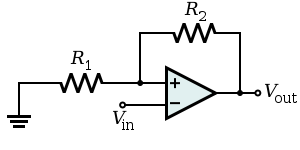Alternatively, the input source and the ground may be swapped. Now the input voltage is applied directly to the inverting input and the non-inverting input is grounded or referenced. The hysteresis curve is inverting and the switching thresholds are$\pm \frac{R_1}{R_1 + R_2} V_{\text{sat}}$. Such a configuration is used in the relaxation oscillator shown below.

### Relaxation oscillator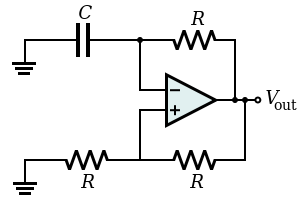By using an RC network to add slow negative feedback to the inverting Schmitt trigger, a relaxation oscillator is formed. The feedback through the RC network causes the Schmitt trigger output to oscillate in an endless symmetric square wave (i.e., the Schmitt trigger in this configuration is an astable multivibrator).

### Inductance gyrator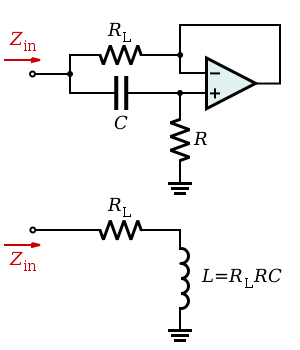Simulates an inductor (i.e., provides inductance without the use of a possibly costly inductor). The circuit exploits the fact that the current flowing through a capacitor behaves through time as the voltage across an inductor. The capacitor used in this circuit is smaller than the inductor it simulates and its capacitance is less subject to changes in value due to environmental changes.

This circuit is unsuitable for applications relying on the back EMF property of an inductor as this will be limited in a gyrator circuit to the voltage supplies of the op-amp.

### Zero level detector

Voltage divider reference

• Zener sets reference voltage.
• Acts as a comparator with one input tied to ground.
• When input is at zero, op-amp output is zero (assuming split supplies.)

### Negative impedance converter (NIC)Creates a resistor having a negative value for any signal generator

• In this case, the ratio between the input voltage and the input current (thus the input resistance) is given by:$R_{\text{in}} = -R_3 \frac{R_1}{R_2}$

In general, the components R1, R2, and R3 need not be resistors; they can be any component that can be described with an impedance.

### Wien bridge oscillatorProduces a very low distortion sine wave. Uses negative temperature compensation in the form of a light bulb or diode.

### Precision rectifier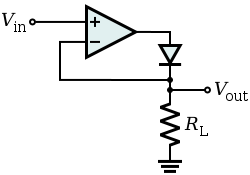The voltage drop VF across the forward biased diode in the circuit of a passive rectifier is undesired. In this active version, the problem is solved by connecting the diode in the negative feedback loop. The op-amp compares the output voltage across the load with the input voltage and increases its own output voltage with the value of VF. As a result, the voltage drop VF is compensated and the circuit behaves very nearly as an ideal (super) diode with VF = 0 V.

The circuit has speed limitations at high frequency because of the slow negative feedback and due to the low slew rate of many non-ideal op-amps.

### Logarithmic output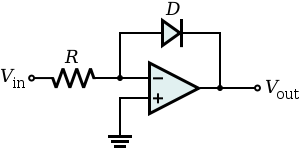• The relationship between the input voltage vin and the output voltage vout is given by:$v_{\text{out}} = -V_{\text{T}} \ln \left( \frac{v_{\text{in}}}{I_{\text{S}} \, R} \right)$
where IS is the saturation current and VT is the thermal voltage.
• If the operational amplifier is considered ideal, the negative pin is virtually grounded, so the current flowing into the resistor from the source (and thus through the diode to the output, since the op-amp inputs draw no current) is:$\frac{v_{\text{in}}}{R} = I_{\text{R}} = I_{\text{D}}$
where ID is the current through the diode. As known, the relationship between the current and the voltage for a diode is:$I_{\text{D}} = I_{\text{S}} \left( e^{\frac{V_{\text{D}}}{V_{\text{T}}}} - 1 \right).$
This, when the voltage is greater than zero, can be approximated by:$I_{\text{D}} \simeq I_{\text{S}} e^{\frac{V_{\text{D}}}{V_{\text{T}}}}.$
Putting these two formulae together and considering that the output voltage is the negative of the voltage across the diode (Vout = − VD), the relationship is proven.

Note that this implementation does not consider temperature stability and other non-ideal effects.

### Exponential output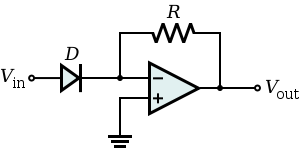• The relationship between the input voltage vin and the output voltage vout is given by:$v_{\text{out}} = -R I_{\text{S}} e^{\frac{v_{\text{in}}}{V_{\text{T}}}}$

where IS is the saturation current and VT is the thermal voltage.

• Considering the operational amplifier ideal, then the negative pin is virtually grounded, so the current through the diode is given by:$I_{\text{D}} = I_{\text{S}} \left( e^{\frac{V_{\text{D}}}{V_{\text{T}}}} - 1 \right)$

when the voltage is greater than zero, it can be approximated by:$I_{\text{D}} \simeq I_{\text{S}} e^{\frac{V_{\text{D}}}{V_{\text{T}}}}.$

The output voltage is given by:$v_{\text{out}} = -R I_{\text{D}}.\,$

## Other applications

Wikimedia Foundation. 2010.

### Look at other dictionaries:

• Operational amplifier — A Signetics μa741 operational amplifier, one of the most successful op amps. An operational amplifier ( op amp ) is a DC coupled high gain electronic voltage amplifier with a differential input and, usually, a single ended output. An op amp… …   Wikipedia

• Current-feedback operational amplifier — Representative schematic of a current feedback op amp or amplifier. The current feedback operational amplifier otherwise known as CfoA or CfA is a type of electronic amplifier whose inverting input is sensitive to current, rather than to voltage… …   Wikipedia

• Operational transconductance amplifier — Der Operationsverstärker (Abk. OP, OPV, OV, OpAmp, OA) ist ein elektronischer Verstärker, der einen invertierenden und einen nichtinvertierenden Eingang besitzt und eine sehr hohe Verstärkung aufweist. Als Eingangsschaltung wird immer ein… …   Deutsch Wikipedia

• Operational transconductance amplifier — Schematic symbol for the OTA. Like the standard operational amplifier, it has both inverting (−) and noninverting (+) inputs; power supply lines (V+ and V−); and a single output. Unlike the traditional op amp, it has two additional biasing inputs …   Wikipedia

• Negative feedback amplifier — Figure 1: Ideal negative feedback model A negative feedback amplifier (or more commonly simply a feedback amplifier) is an amplifier which combines a fraction of the output with the input so that a negative feedback opposes the original signal.… …   Wikipedia

• Fully differential amplifier — A fully differential amplifier, usually referred to as an FDA for brevity, is a DC coupled high gain electronic voltage amplifier with differential inputs and differential outputs. In its ordinary usage, the output of the FDA is controlled by two …   Wikipedia

• Instrumentation amplifier — An instrumentation (or instrumentational) amplifier is a type of differential amplifier that has been outfitted with input buffers, which eliminate the need for input impedance matching and thus make the amplifier particularly suitable for use in …   Wikipedia

• Electronic amplifier — A practical amplifier circuit An electronic amplifier is a device for increasing the power of a signal. It does this by taking energy from a power supply and controlling the output to match the input signal shape but with a larger amplitude. In… …   Wikipedia

• Differential amplifier — symbol The inverting and non inverting inputs are distinguished by − and + symbols (respectively) placed in the amplifier triangle. Vs+ and Vs− are the power supply voltages; they are often omitted from the diagram for simplicity, but of course… …   Wikipedia

• Valve amplifier — A valve amplifier or tube amplifier is a type of electronic amplifier that make use of vacuum tubes instead of solid state semiconductor devices (such as transistors). As any other electronic amplifier they serve to increase the power and/or… …   Wikipedia